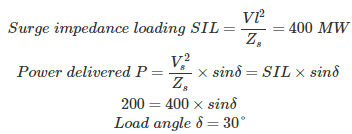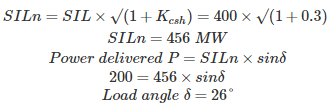# MCQs on Power Systems

##### Page 15 of 67. Go to page 1 2 3 4 5 6 7 8 9 10 11 12 13 14 15 16 17 18 19 20 21 22 23 24 25 26 27 28 29 30 31 32 33 34 35 36 37 38 39 40 41 42 43 44 45 46 47 48 49 50 51 52 53 54 55 56 57 58 59 60 61 62 63 64 65 66 67
01․ A transmission line has a surge impedance of 400 Ω is connected with the cable having surge impedance of 40 Ω, a surge magnitude of 100 kV is travelling from the transmission line towards the cable. Find the reflected voltage?
81.82 kV
18.18 kV
-81.82 kV
-18.18 kV

Transmitted voltage V" = V + V' Transmitted current I" = I + I' Transmission or reflection coefficient of voltage Tv = V"/V Transmission or reflection coefficient of current Ti = I"/I I" = V"/Zl, I = V/Zs, I' = -V'/Zs V"/Zl = V/Zs - V'/Zs V' = V" - V Therefore, Tv = V"/V = 2Zl/(Zl + Zs) Reflection coefficient of voltage is Rv= V'/V = Tv - 1 Rv = V'/V = (Zl - Zs)/(Zs + Zl)02․ Surge impedance loading of a line is increased by which of the following compensation methods?
shunt capacitor
series capacitor
shunt reactor
both 1 and 2

03․ A transmission line has surge impedance of 400 Ω, operating at Vs = Vr = 400 kV line is compensated with a shunt capacitance of 30%. Find the surge impedance loading with compensation?
400 MW
478 MW
456 MW
386 MW

Surge impedance loading with out compensation SIL = Vl²/Zs = (400*10³)²/400 = 400 MW Surge impedance loading with shunt capacitance compensation Shunt capacitor compensation: After shunt capacitor compensation new surge impedance Zn = √(L/C(1 + Kcsh)) Where Kcsh = Degree of shunt capacitance = Csh/C Therefore, new surge impedance Zn = Zs/√(1 + Kcsh) New surge impedance loading SILn = SIL*√(1 + Kcsh) SILn = 400*√(1+0.3) =456 MW

04․ A transmission line has surge impedance of 400 Ω, operating at Vs = Vr = 400 kV line is compensated with a shunt capacitance of 30%. The line is connected to a generator delivering 200 MW. Find the load angle with out and with compensation respectively?
30° and 45°
30° and 26°
26° and 30°
45° and 26°

With out compensation:With compensation:05․ If the load is short circuited, then reflection coefficient of voltage is
-1
1
0
-2

Transmission or reflection coefficient of voltage Tv = V"/V Transmission or reflection coefficient of current Ti = I"/I I" = V"/Zl, I = V/Zs, I' = -V'/Zs V"/Zl = V/Zs - V'/Zs V' = V" - V Therefore, Tv = V"/V = 2Zl/(Zl + Zs) Reflection coefficient of voltage is Rv = Tv - 1 Rv = (Zl - Zs)/(Zs + Zl) When load is short circuited, V" = 0 Therefore, Tv = 0 and Rv = -1

06․ The Y bus matrix of a 100 bus interconnected system is 90% sparse. Then find the number of non zero elements in the Y bus matrix?
9000
90
1000
10

Sparsity of Y bus matrix = Number of zero elements/ Total number of elements Total number of elements for 100 bus = 100*100 = 10000 Number of zero elements = 0.9 * 10000 =9000 Therefore, number of non zero elements = 1000

07․ At slack bus, which one of the following combinations of variables is not specified?
P and V
P and Q
V and δ
Q and V

Load bus: Known quantities - P and Q Unknown quantities - V and δ Generator bus: Known quantities - P and V Unknown quantities - Q and δ Slack or reference bus: Known quantities - V and δ Unknown quantities - P and Q

08․ Which of the following statements are true?
Bus admittance matrix is a sparse matrix
Bus impedance matrix is a full matrix
both 1 and 2
nether 1 nor 2

Y bus matrix is a sparse matrix, containing more number of zero elements. So that faster calculation is possible. The Y bus matrix is used for the load flow studies. Z bus algorithm or matrix is used for the fault analysis. Inverse of Y bus matrix gives the Z bus matrix, But Z matrix is a full matrix even though Y bus is a sparse matrix. More time is required for inverse of Z bus matrix if the size of the matrix is more than three. But a practical system network has large number of buses and hence Z bus matrix is not uses for the load flow analysis, Y bus is preferred.

09․ Which of the following statements is/are true? The elements of each row of a Y bus matrix for load flow studies in power system add up to zero
always
if the shunt admittances at the buses are ignored
if the mutual couplings between transmission lines are absent
if both 2 and 3 are satisfied

Properties of Y bus matrix: 1. Y bus is a square matrix. 2. For n bus power system size of Y bus matrix is n*n 3. Value of diagonal element corresponding to bus i, then Yii = Sum of the admittances connected to bus i. 4. The value of off diagonal elements Yij = Yji , which is connected between bus i and bus j and which is represented with negative sign. 5. Sum of the elements of row i equal to shunt admittances connected to bus i. If this summation is zero, indicates there is no shunt admittance and mutual coupling between the transmission lines.

10․ In a Gauss Seidel load flow method, the number of iterations may be reduced if the correction in voltage at each bus is multiplied by
Gauss constant
Acceleration factor
Blocking factor
Lagrange multiplier

Advantages of gauss siedel method: 1. It is a simple algebraical equation, so that calculation time for each iteration is less. 2. More suitable for small size networks. Disadvantages of gauss siedel method: 1. More number of iterations are required, so that it has slow convergence. 2. Initial approximate guessing value is required for convergence. 3. It required accelerating factor for convergence. 4. The choice of slack bus affects the convergence. 5. It is not applicable for the large power system networks. Accelerating factor is used for reducing number of iterations using Gauss Seidel method. The value of accelerating factor is around 1.6 to 1.8 .

<<<1314151617>>>